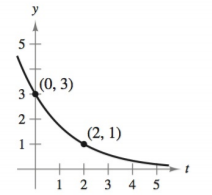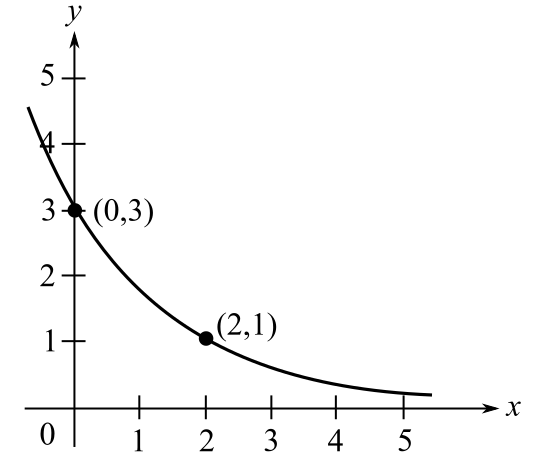Chapter 4.6, Problem 4E### Calculus: An Applied Approach (Min...

10th Edition
Ron Larson
ISBN: 9781305860919

#### Solutions

Chapter
Section### Calculus: An Applied Approach (Min...

10th Edition
Ron Larson
ISBN: 9781305860919
Textbook Problem
1 views

# Modeling Exponential Growth and Decay In Exercises 1-6, find the exponential function y = C e k t that passes through the two given points. y = C e k tTo determine

To calculate: The exponential function y=Cekt for the following graph:Explanation

Given Information:

The provided graph is:

Consider the following graph:

Observe that the exponential function curve passes through the points (0,3) and (2,1).

Hence the points satisfy the equation of exponential function y=Cekt.

Substitute t=0,y=3 in the equation y=Cekt.

3=Cek(0)3=Ce03=C1C=3

Hence, the value of C is 3.

Substitute t=2,y=1 in the equation y=3ekt.

1=3ek(2)1=3e2k

Solve the equation 1=3e2k for k.

e2k=13

Taking natural logarithm of both the sides

### Still sussing out bartleby?

Check out a sample textbook solution.

See a sample solution

#### The Solution to Your Study Problems

Bartleby provides explanations to thousands of textbook problems written by our experts, many with advanced degrees!

Get Started

#### Determine whether f'(0) exists. f(x)={x2sin1xifx00ifx=0

Single Variable Calculus: Early Transcendentals, Volume I

#### In Exercises 7-12, refer to the following figure. 8. What are the coordinates of point B?

Applied Calculus for the Managerial, Life, and Social Sciences: A Brief Approach

#### In the problems 7-8, find the intercept and graph the functions 5x6y=30

Mathematical Applications for the Management, Life, and Social Sciences

#### Find the limit. limx0sin(x2)x

Single Variable Calculus: Early Transcendentals

#### The polar form for the graph at the right is:

Study Guide for Stewart's Multivariable Calculus, 8th

#### What is the general purpose of a manipulation check?

Research Methods for the Behavioral Sciences (MindTap Course List)